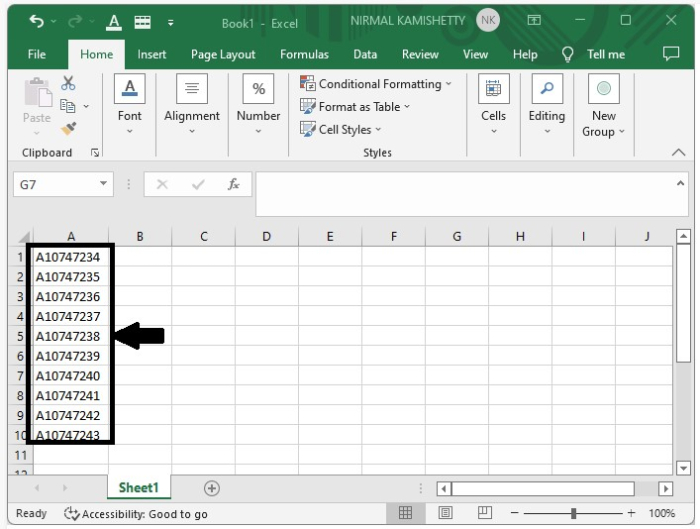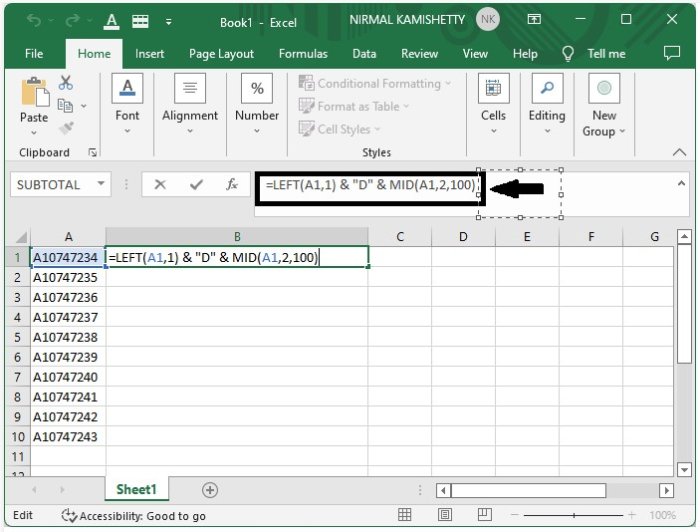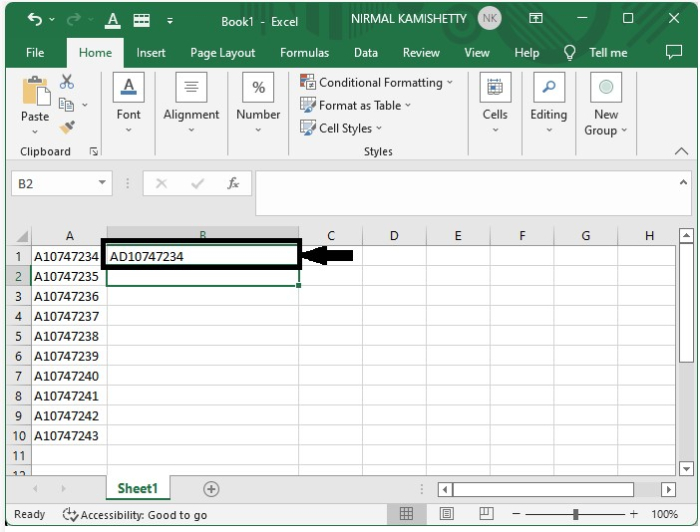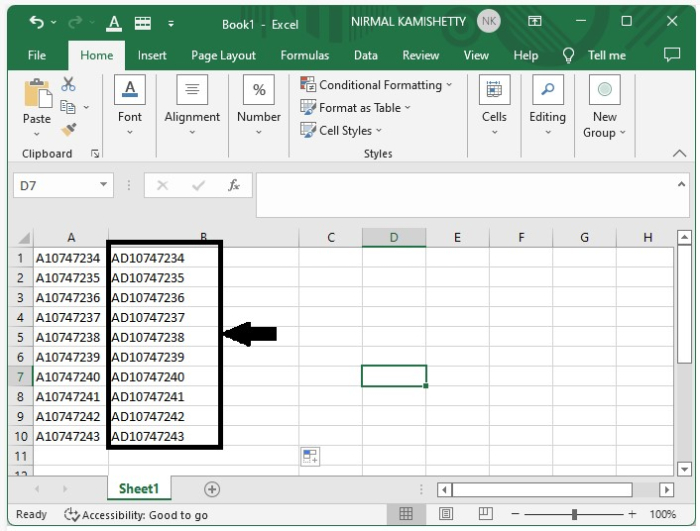# How to Add Text in the Middle of Selected Cells in Excel?

Suppose you have an Excel sheet with a column of data and you need to add a string of text at a specific position in all the column data. In such cases, you don't have to type the entire data all over again to update the given column data. In this tutorial, we will demonstrate in a few simple steps how you can add the new data in between an existing set of data.

## Add Text in the Middle of Selected Cells in Excel

Suppose we have an Excel sheet with a list of data that starts with the letter "A" and the sequence is in the order "A10747234". And, we want to change the series of data to "AD10747234". Then, we can use a formula to add a "D" to the sequence for all the given data.

### Step 1

Open an Excel sheet and insert a sequence of data, as shown below −### Step 2

Now, click the cell where you want to show the updated data, and insert the formula =LEFT(A2,1) & "D" & MID(A2,2,100) in the formula box −Press "Enter" to get the first result.### Step 3

After getting the first result in the selected cell, just drag it from the right corner to get all the other results.This is how you can insert the data between the text in selected cells.

## Tips to Understand the Formula Used

In our example, we have used the following formula

=LEFT(A2,1) & "D" & MID(A2,2,100)

Here "A2" represents the address of the data where changes needed to be made. "1" represents the data inserted after one character in the data.

"D" is the date that we want to insert. "MID" represents the middle. "100" represents the end characters of the data.

We can change the way of inserting the data just by changing the formula.